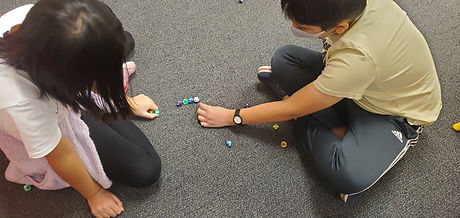## Mr. Jake

### Target 1​

###### Lesson Type:

Continuation

Critical Thinking

:

Logical Reasoning

Use reasoning to solve logic puzzles.

###### 1:

Use the given information in a problem to identify the correct answer.

###### 2:

Follow given rules to solve problems.

###### 3:

Think outside of the box to solve problems.

7th

###### Vocabulary:

Tower of Hanoi, Optimal

Activities:

Found the optimal solution for different versions of the tower of Hanoi problem and the pattern/equation that determines the number of moves for the optimal solution### Home Exploration

###### Guiding Questions:## Absent Students:

### Target 2

:

###### 1:

Construct and read box and whisker plots.

###### 2:

Identify the parts of a box and whisker plot (median, quartile, upper quartile, lower quartile, and extremes).

###### 3:

Define median (the middle of a set of data), quartile (dividing data into four sections), upper quartile (the median of a quartile on the higher end of the range), lower quartile (the median of a quartile on the lower range), and extremes (the highest and lowest scores in the data range).

###### 4:

Draw a box and whisker plot to represent given data.

###### 5:

Order a set a data to find the median, quartiles, and extremes (minimum and maximum).

HS

###### Vocabulary:

Box and Whisker Plot, Data, Distribution, Quartile, Median, Minimum, Maximum

Activities:

Collected data and created box and whisker plots to observe the distribution of the data### Home Exploration

###### Guiding Questions:### Target 3

:

###### Vocabulary:

Activities:### Home Exploration